# Motion And Its Types- Download Science Study Notes Free PDF For REET ExamIn many teaching exams including  REETUTET DSSSB Exams etc. Science may be an interesting subject with interesting notes and quizzes. In TET Exams, there will be 30 questions i.e. 15 Questions Science content and 15 questions of Science Pedagogy in REETUTET  and other State TET Exams. Science comprising of various branches of studies like chemistry, physical science, and life science.

Get free Study material for REET Exam

## MOTION AND ITS TYPES

Motion:
An object is said to be in motion, if its position changes with respect to its surroundings in given time. If the position of an object does not change with the time, it is said to be at rest. e.g. A car speeding on road, a ship on water, the movement of a snail on the ground, a butterfly flitting from flower to flower, moon going around the earth are the examples of motion.
When an object or body repeats its motion after some period of time then it is said to be in periodic motion. e.g. Motion of the earth around the sun, motion of the moon around the earth, etc.

#### How To Score 25+ in Science For CTET Exam

Types of Motion:
(i) Rectilinear Motion: Rectilinear motion is that motion in which a particle or body is moving along a straight line. e.g. : A car moving on a straight road.
(ii) Circular Motion: A circular motion is that motion in which a particle or body is moving in a circle. Circular motion can be two – dimensional or three – dimensional. It is also a periodic motion. e.g. The motion of a point marked on the blade of an electric fan or the hands of a clock.
(iii) Oscillatory Motion: Oscillatory motion is that motion in which a body moves to and fro or back and forth repeatedly about a fixed point in a definite interval of time. This type of motion is also a type of periodic motion, e.g. A swing.

 Examples of different types of Motion Soldiers in a march past Rectilinear motion Bullock cart moving on a straight road Rectilinear motion Pedal of a bicycle in motion Circular motion Motion of a swing Oscillatory motion Motion of a pendulum Oscillatory motion

Practice REET Previous Year EVS Quiz For REET Exam Here

Terms Related to Motion:
• Distance: The length of the actual path covered by a body in a time interval is called distance. Distance is a scalar quantity, which has magnitude only.

• Odometer: is an instrument used to measure distance in vehicle.

• Displacement: The difference between the final and the initial position of an object is called displacement. Displacement is a vector quantity, which has both magnitude and direction.
If a body is rotating in a circular path, then after one rotation its displacement will be zero but the distance traveled will be equal to the circumference of the circle.

• Speed: The distance covered by an object in unit interval of time is called the speed of the object.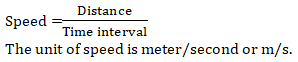• Average Speed: The average speed of a particle for a given interval of time is defined as the ratio of total distance traveled to the time taken.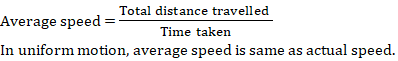• Velocity: The displacement traveled by an object in a unit interval of time is called the velocity of object.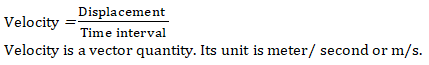Speed is always equal to or greater than magnitude of the velocity.

• Average Velocity: The average velocity of a particle for a given interval of time is defined as the ratio of total displacement traveled to the time taken.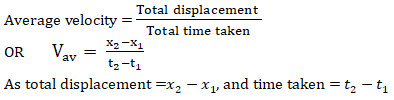• Relative Velocity: The rate of change of position of a body with reference to moving observer is regarded as the relative velocity of the body w.r.t. observer.
When both the observer and the object to be observed are moving in same direction then, relative velocity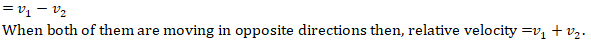Check Complete Science Study Now: HERE

• Acceleration: The rate of change of velocity of an object is called the acceleration of that object.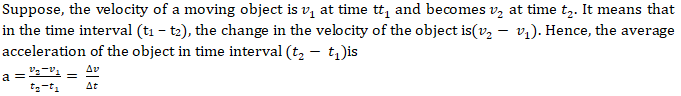The unit of acceleration is meter/second² or m/s².

• If the velocity of an object increases without change indirection, it is said to be moving with positive acceleration.
• If the velocity of an object decreases without change in direction, the object is said to be moving with negative acceleration or deceleration or retardation.
• An object is said to be moving with uniform acceleration if its velocity changes by equal amounts in equal intervals of time.
• An object is said to be moving with a variable acceleration if its velocity changes by unequal amounts in equal intervals of time.
• Acceleration of an object is zero if it is at rest or is moving with uniform velocity.

Classification of Motion:
1. Uniform Motion: An object moving along a straight line with a constant speed is said to be in uniform motion.
2. Non–Uniform Motion: Motion If the speed of an object moving along a straight line keeps changing its motion then the body is said to be in non – uniform motion.

Graphical Representation of Motion:

I. Distance – Time Graph:
• The nature of the motion of an object can be studied by plotting a graph between the distance covered and time. Such a graph is called a distance – time graph. .
• The distance – time graph of an object moving with a uniform speed is a straight line.
• If an object moves with a non – uniform speed, its distance – time graph is not a straight line. The inclination of the graph is different at different places and hence, it does not have a unique slope.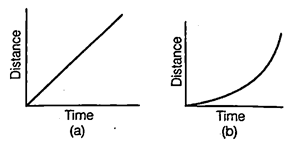(a) Distance – time graph for uniform speed
(b) Distance – time graph for non – uniform speed

II. Displacement – Time Graph:
• The displacement – time graph of an object moving with a uniform velocity is a straight line. The slope of the displacement – time graph of an object equal to its velocity.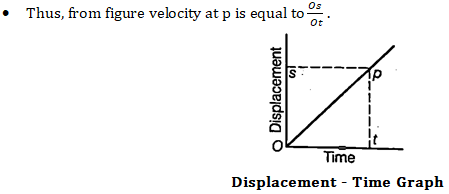III. Speed – Time Graph:
• If an object moves with a constant speed, its speed – time graph is a straight line parallel to the time axis.
• The area under the speed – time graph gives the distance traversed by the object in the corresponding time interval.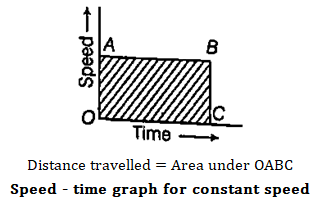IV. Velocity – Time Graph
• The slope of the velocity – time graph gives the acceleration the object
• If an object moves with a constant acceleration in a straight line, its velocity – time graph is a straight line.
• The area under a velocity – time graph gives the displacement of the object.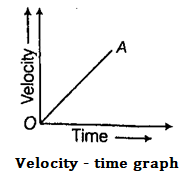Equations of Motion:
1. First Equation of Motion: If a body having initial velocity u is subjected to a uniform acceleration a, then after time t, its final velocity v, is
v = u + at
2. Second Equation of Motion: The distance covered by a body in time t moving with initial velocity u and acceleration a, is

s = ut +   at²

3. Third Equation of Motion: If a body having initial velocity u, final velocity v, acceleration a travels a distance s1 then

v² = u² + 2as

Motion of Body under Gravity:

The force of attraction of earth on bodies is called force of gravity. Acceleration produced in the body by the force of gravity is called acceleration due to gravity. It is represented by g. Thus,

If a body is projected vertically downward with some Initial velocity, then equation of motion is

v = u + gt

b = ut +  gt²

v² = u² + 2gh

If a body is projected vertically upward, then equation of motion is

v = u – gt

b = ut –  gt²

v² = u² = 2gh

Where, h is the height of the body, u is initial velocity, v is final velocity and t is time interval for the vertical motion.

Sharing is caring!

×

Thank You, Your details have been submitted we will get back to you.Join India's largest learning destination

What You Will get ?

•Job Alerts
•Daily Quizzes
•Subject-Wise Quizzes
•Current Affairs
•Previous year question papers
•Doubt Solving session

ORJoin India's largest learning destination

What You Will get ?

•Job Alerts
•Daily Quizzes
•Subject-Wise Quizzes
•Current Affairs
•Previous year question papers
•Doubt Solving session

ORJoin India's largest learning destination

What You Will get ?

•Job Alerts
•Daily Quizzes
•Subject-Wise Quizzes
•Current Affairs
•Previous year question papers
•Doubt Solving session

Enter the email address associated with your account, and we'll email you an OTP to verify it's you.Join India's largest learning destination

What You Will get ?

•Job Alerts
•Daily Quizzes
•Subject-Wise Quizzes
•Current Affairs
•Previous year question papers
•Doubt Solving session

Enter OTP

Please enter the OTP sent to
/6

Did not recive OTP?

Resend in 60sJoin India's largest learning destination

What You Will get ?

•Job Alerts
•Daily Quizzes
•Subject-Wise Quizzes
•Current Affairs
•Previous year question papers
•Doubt Solving sessionJoin India's largest learning destination

What You Will get ?

•Job Alerts
•Daily Quizzes
•Subject-Wise Quizzes
•Current Affairs
•Previous year question papers
•Doubt Solving session

Almost there

+91Join India's largest learning destination

What You Will get ?

•Job Alerts
•Daily Quizzes
•Subject-Wise Quizzes
•Current Affairs
•Previous year question papers
•Doubt Solving session

Enter OTP

Please enter the OTP sent to Edit Number

Did not recive OTP?

Resend 60

By skipping this step you will not recieve any free content avalaible on adda247, also you will miss onto notification and job alerts

Are you sure you want to skip this step?

By skipping this step you will not recieve any free content avalaible on adda247, also you will miss onto notification and job alerts

Are you sure you want to skip this step?